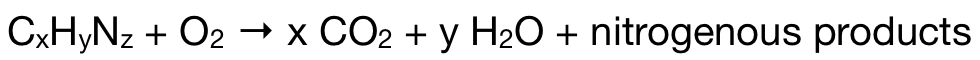# Problem: An organic compound containing only C, H, and N yields the following data.i. Complete combustion of 35.0 mg of the compound produced 33.5 mg CO2 and 41.1 mg H2O.ii. A 65.2-mg sample of the compound was analyzed for nitrogen by the Dumas method (see Exercise 129), giving 35.6 mL of dry N2 at 740. torr and 25°C.iii. The effusion rate of the compound as a gas was measured and found to be 24.6 mL/min. The effusion rate of argon gas, under identical conditions, is 26.4 mL/min. What is the molecular formula of the compound?

###### FREE Expert Solution

The formula for the unknown compound is CxHyNz. The combustion reaction of the unknown is:From this, we can see that all of the CO2 and H2O formed came from the unknown. Complete combustion of 35.0 mg of the unknown produced 33.5 mg CO2 and 41.1 mg H2O

93% (143 ratings)###### Problem Details

An organic compound containing only C, H, and N yields the following data.
i. Complete combustion of 35.0 mg of the compound produced 33.5 mg CO2 and 41.1 mg H2O.
ii. A 65.2-mg sample of the compound was analyzed for nitrogen by the Dumas method (see Exercise 129), giving 35.6 mL of dry N2 at 740. torr and 25°C.
iii. The effusion rate of the compound as a gas was measured and found to be 24.6 mL/min. The effusion rate of argon gas, under identical conditions, is 26.4 mL/min. What is the molecular formula of the compound?